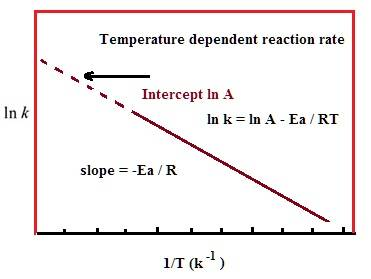# Relationship between ln k and 1/t using different formula

#### Ethan Cheng

Hi, I'm currently taking Chemistry 101 and came across this equation that seems to contradict what I've learned before. I don't know the name of it, but here is the equation and its implication.Now another equation we have learned is the Arrhenius equation, which is as follows:If I understood the equations correctly, they are referring to the same k (equilibrium constant) and the same t (temperature), which gave rise to something that seems to be wrong.

Take the example of combustion, where the activation energy is above 0 and is an exothermic reaction. The graph produced by the first equation will be one with a positive slope, but the one produced by the second equation is one with a negative slope, even though they have the same axis. To illustrate this further, if we somehow experimentally determined the ln k and 1/t (appears very often in our homework), we usually start by graphing them. The linear regression is seen (using Arrhenius equation) as -Ea/R, and we can, therefore, use it to find activation energy. But this same slope should also be -H / R, and therefore will lead to Ea = H, which is also obviously wrong.

What am I missing here? Are the K referring to different values?

#### Attachments

• 392.2 KB Views: 114
• 349.8 KB Views: 113
• 23.2 KB Views: 227

#### Chestermiller

Mentor
The equation in your first figures refers to the equilibrium constant and the heat of reaction. The equation in your 2nd figure refers to the reaction rate constant and the activation energy for the reaction.

#### Borek

Mentor
Are the K referring to different values?
Yes. One is often written as k (for kinetics), the other as K (for equilibrium).

### The Physics Forums Way

We Value Quality
• Topics based on mainstream science
• Proper English grammar and spelling
We Value Civility
• Positive and compassionate attitudes
• Patience while debating
We Value Productivity
• Disciplined to remain on-topic
• Recognition of own weaknesses
• Solo and co-op problem solving